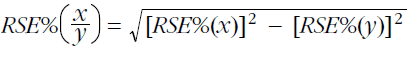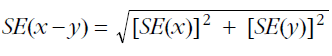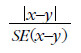6553.0 - Survey of Income and Housing, User Guide, Australia, 2013-14
Previous ISSUE Released at 11:30 AM (CANBERRA TIME) 04/09/2015
 Page tools: .mffeedback,#pjs { display :none; } Enable Javascript to Print PagesPrint AllEmail NotificationRSSSearch this Product RELIABILITY OF ESTIMATES SAMPLING VARIABILITY The estimates provided from the Survey of Income and Housing (SIH) are subject to two types of error, non-sampling and sampling error. Comparisons between estimates from surveys conducted in different periods, for example, comparison of 2013–14 SIH estimates with previous cycle estimates, are also subject to the impact of any changes made to the way the survey is conducted. For further details on changes between cycles see the 'Historical information' section of this publication. Non-sampling error Non-sampling error can occur in any collection, whether the estimates are derived from a sample or from a complete collection such as a census. Sources of non-sampling error include non-response, errors in reporting by respondents or recording of answers by interviewers and errors in coding and processing the data. Non-sampling errors are difficult to quantify in any collection. However, every effort is made to reduce non-sampling error to a minimum by careful design and testing of the questionnaire, training of interviewers and data entry staff, and extensive editing and quality control procedures at all stages of data processing. One of the main sources of non-sampling error is non-response by persons selected in the survey. Non-response occurs when people cannot or will not cooperate or cannot be contacted. Non-response can affect the reliability of results and can introduce a bias. The magnitude of any bias depends upon the level of non-response and the extent of the difference between the characteristics of those people who responded to the survey and those who did not. The following methods were adopted to reduce the level and impact of non-response: Primary Approach Letters (PALs) were posted to selected SIH households prior to enumeration; document cards were provided to respondents to suggest having financial statements and similar documents handy at the time of interview to assist with accurate responses; face-to-face interviews with respondents; the use of interviewers who could speak languages other than English, where necessary; Proxy Interviews conducted, when consent is given, with a responsible person answering on behalf of respondents incapable of doing so themselves; follow-up of respondents if there was initially no response; imputation of missing values; and ensuring that the weighted data is representative of the population (in terms of demographic characteristics) by aligning the estimates with population benchmarks. Sampling error The estimates are based on a sample of possible observations and are subject to sampling variability. The estimates may therefore differ from the figures that would have been produced if information had been collected for all households. A measure of the sampling error for a given estimate is provided by the standard error, which may be expressed as a percentage of the estimate. The estimates from the SIH are based on information obtained from the occupants of sampled dwellings. Therefore, the estimates are subject to sampling variability and may differ from the population parameters that would have been observed if information had been collected for all dwellings. One measure of the likely uncertainty is given by the standard error estimate (SE), which indicates the extent to which a sample estimate might have varied compared to the population parameter because only a sample of dwellings were included. There are about two chances in three that the sample estimate will differ by less than one SE from the population parameter that would have been obtained if all dwellings had been enumerated, and about 19 chances in 20 that the difference will be less than two SEs. Another measure of the likely difference is the relative standard error (RSE), which is obtained by expressing the SE as a percentage of the estimate. For estimates of population sizes, the size of the SE generally increases with the level of the estimate, so that the larger the estimate the larger the SE. However, the larger the sampling estimate the smaller the SE becomes in percentage terms. Thus, larger sample estimates will be relatively more reliable than smaller estimates. Estimates with RSEs of 25% or more are not considered reliable for most purposes. Estimates with RSEs greater than 25% but less than or equal to 50% are annotated by an asterisk to indicate they are subject to high SEs and should be used with caution. Estimates with RSEs of greater than 50%, annotated by a double asterisk, are considered too unreliable for general use and should only be used to aggregate with other estimates to provide derived estimates with RSEs of 50% or less. RSEs for the SIH have been derived using the delete-a-group jackknife method. If needed, SEs can be calculated using the estimates and RSEs. MEASURES OF RELIABILITY Proportions and percentages Proportions and percentages, which are formed from the ratio of two estimates, are also subject to sampling errors. The size of the error depends on the accuracy of both the numerator and the denominator. For proportions where the denominator is an estimate of the number in a grouping and the numerator is the number in a subgroup of the denominator group, the formula for an approximate RSE is given by:The RSE estimates for proportions listed in SIH published output calculate the effect of correlations between the numerator and the denominator. Differences between estimates The difference between survey estimates is also subject to sampling variability. An approximate SE of the difference between two estimates (x–y) may be calculated by the formula:This approximation can generally be used whenever the estimates come from different samples, such as two estimates from different years or two estimates for two non-intersecting subpopulations in the one year. If the estimates come from two populations, one of which is a subpopulation of the other, the standard error is likely to be lower than that derived from this approximation. Significance testing When comparing estimates between surveys or between populations within a survey, it is useful to determine whether apparent differences are 'real' differences between the corresponding population characteristics or simply the result of sampling variability between the survey samples. One way to examine this is to determine whether the difference between the estimates is statistically significant. This is done by calculating the standard error of the difference between two estimates (x and y), see the 'Differences between estimates' section above, and applying that to calculate the test statistic using the formula below:If the value of this test statistic is greater than 1.96 (at the 95% confidence level) then there is good evidence of a statistically significant difference between the two population estimates with respect to that characteristic. Otherwise, it cannot be stated with confidence that there is a real difference between the population estimates. Document Selection These documents will be presented in a new window.